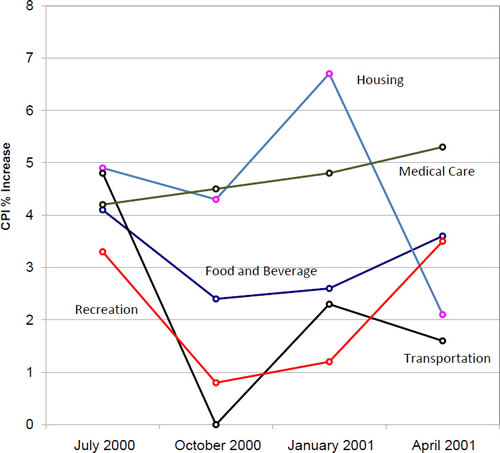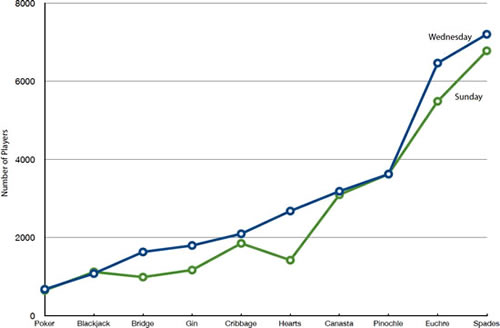# 2.9: Line Graphs

•• Contributed by David Lane
• Associate Professor (Psychology, Statistics, and Management) at Rice University

Skills to Develop

• Create and interpret line graphs
• Judge whether a line graph would be appropriate for a given data set

A line graph is a bar graph with the tops of the bars represented by points joined by lines (the rest of the bar is suppressed). For example, Figure $$\PageIndex{1}$$ was presented in the section on bar charts and shows changes in the Consumer Price Index (CPI) over time.Figure $$\PageIndex{2}$$: A line graph of the percent change in the CPI over time. Each point represents percent increase for the three months ending at the date indicated.

A line graph of these same data is shown in Figure $$\PageIndex{2}$$. Although the figures are similar, the line graph emphasizes the change from period to period.

Line graphs are appropriate only when both the $$X$$- and $$Y$$-axes display ordered (rather than qualitative) variables. Although bar graphs can also be used in this situation, line graphs are generally better at comparing changes over time. Figure $$\PageIndex{3}$$, for example, shows percent increases and decreases in five components of the Consumer Price Index (CPI). The figure makes it easy to see that medical costs had a steadier progression than the other components. Although you could create an analogous bar chart, its interpretation would not be as easy.Figure $$\PageIndex{3}$$: A line graph of the percent change in five components of the CPI over time.

Let us stress that it is misleading to use a line graph when the $$X$$-axis contains merely qualitative variables. Figure $$\PageIndex{4}$$ inappropriately shows a line graph of the card game data from Yahoo, discussed in the section on qualitative variables. The defect in Figure $$\PageIndex{4}$$ is that it gives the false impression that the games are naturally ordered in a numerical way.Figure $$\PageIndex{4}$$: A line graph, inappropriately used, depicting the number of people playing different card games on Sunday and Wednesday.

## Contributors

• Online Statistics Education: A Multimedia Course of Study (http://onlinestatbook.com/). Project Leader: David M. Lane, Rice University.